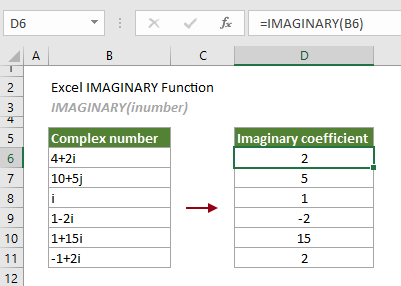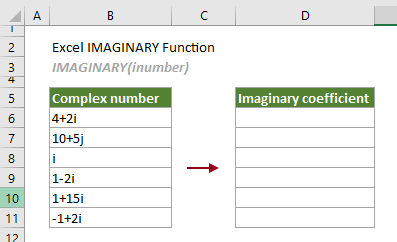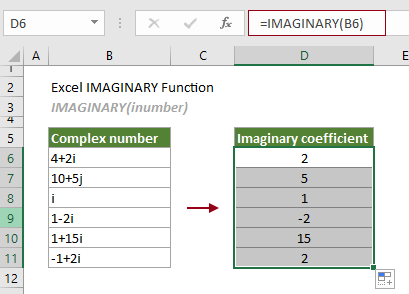## Excel IMAGINARY function

The IMAGINARY function returns the imaginary coefficient of a given complex number in the form x + yi or x + yj.

Note: A complex number is composed of a real coefficient and an imaginary coefficient. You can apply the COMPLEX function to convert real and imaginary coefficients to a complex number of the form x + yi or x + yj.#### Syntax

IMAGINARY(inumber)

#### Arguments

• Inumber (required): The complex number for which you want to return the imaginary coefficient.

#### Remarks

1. The argument can be supplied as any of the following:
-- A real number whose imaginary part is 0, e.g., 1 is a complex number 1+0i; Or a purely imaginary number whose real part is 0, e.g., i is a complex number 0 + 1i;
-- A cell reference that refers to a complex number;
-- A complex number enclosed in double quotation marks.
2. The #VALUE! error occurs if “inumber” is a logical value.
3. The #NUM! error occurs if “inumber” cannot be recognized as a complex number.

#### Return value

It returns a numeric value.

#### Example

In the following table, there is a list of complex numbers. To get the imaginary coefficients of these complex numbers, you can apply the IMAGINARY function as follows to get it done.Select a cell (says D6 in this case), enter the formula below and press the Enter key to get the first imaginary coefficient. Select this result cell and then drag its AutoFill Handle down to get other results.

=IMAGINARY(B6)Notes:

1) The complex number can be directly entered into the formula and needs to be enclosed in double quotation marks. So, the formula in D6 can be changed to:

=IMAGINARY("4+2i")

2) The IMAGINARY function accepts only lowercase “i” and “j”.

#### Related Functions

Excel COMPLEX function
The COMPLEX function converts real and imaginary coefficients to a complex number of the form x + yi or x + yj.

Excel IMABS function
The IMABS function gets the absolute value of a complex number in the form x + yi or x + yj.

Excel impower function
The IMPOWER function returns a complex number raised to a given power.

Excel improduct Function
The IMPRODUCT function returns the product of 1 to 255 complex numbers in x + yi or x + yj text format.

Excel BITAND function
The IMREAL function returns the real coefficient of a complex number in the form x + yi or x + yj.

Excel IMSUB function
The IMSUB function returns the difference of two complex numbers in x + yi or x + yj text format.

Excel IMSUM function
The IMSUM function returns the sum of two or more complex numbers in x + yi or x + yj text format.

### The Best Office Productivity Tools

#### Kutools for Excel - Helps You To Stand Out From Crowd

 Popular Features: Find, Highlight or Identify Duplicates  |  Delete Blank Rows  |  Combine Columns or Cells without Losing Data  |  Round without Formula ... Super VLookup: Multiple Criteria  |  Multiple Value  |  Across Multi-Sheets  |  Fuzzy Lookup... Adv. Drop-down List: Easy Drop Down List  |  Dependent Drop Down List  |  Multi-select Drop Down List... Column Manager: Add a Specific Number of Columns  |  Move Columns  |  Toggle Visibility Status of Hidden Columns  |  Compare Columns to Select Same & Different Cells ... Featured Features: Grid Focus  |  Design View  |  Big Formula Bar  |  Workbook & Sheet Manager | Resource Library (Auto Text)  |  Date Picker  |  Combine Worksheets  |  Encrypt/Decrypt Cells  |  Send Emails by List  |  Super Filter  |  Special Filter (filter bold/italic/strikethrough...) ... Top 15 Toolsets:  12 Text Tools (Add Text, Remove Characters ...)  |  50+ Chart Types (Gantt Chart ...)  |  40+ Practical Formulas (Calculate age based on birthday ...)  |  19 Insertion Tools (Insert QR Code, Insert Picture from Path ...)  |  12 Conversion Tools (Numbers to Words, Currency Conversion ...)  |  7 Merge & Split Tools (Advanced Combine Rows, Split Excel Cells ...)  |  ... and more

Kutools for Excel Boasts Over 300 Features, Ensuring That What You Need is Just A Click Away...#### Office Tab - Enable Tabbed Reading and Editing in Microsoft Office (include Excel)

• One second to switch between dozens of open documents!
• Reduce hundreds of mouse clicks for you every day, say goodbye to mouse hand.
• Increases your productivity by 50% when viewing and editing multiple documents.
• Brings Efficient Tabs to Office (include Excel), Just Like Chrome, Edge and Firefox.